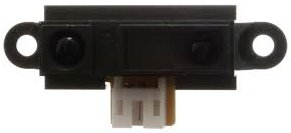# How to Build an Infrared Distance Sensor Circuit

An infrared (IR) distance sensor is a sensor that can measure distance from a solid or physical object.An infrared distance sensor does this through light waves.
It sends out infrared light and waits to receive it back at the receiver end. If it receives back the infrared light, this means that the light waves have bounced off of a physical object and has returned back to the sensor. If it does not receive the light waves back, then this means there is no physical object in front of it within viewing angle.
The infrared sensor we will use is the Sharp GP2Y0A21 Infrared Distance Sensor.
We will write code so that if the sensor detects an object that is within a few inches of it, it will turn on and light up an LED.
This is an analog output LED, so we will connect this sensor to an arduino microcontroller in order to read the analog output. Therefore, we will connect the sensor to an Arduino microcontroller to build this infrared distance sensor circuit.

### Components

• GP2Y0A21 Infrared Distance Sensor
• LED
• Arduino

The Sharp GP2Y0A21 Ultrasonic Distance Sensor is relatively cheap. It can be gotten for a little over \$6 on ebay.
It runs on anywhere from 4.5V- 5.5V of DC power, so the 5V terminal that the arduino has is perfect for powering this infrared sensor.
The sensor typically runs on 30mA of current. So it uses about 150mW (30mA x 5V= 150mW).
It has a pretty quick response time of 30ms (±10).
The datasheet of the sensor can be found at the following link: GP2Y0A21 Infrared Distance Sensor Datasheet.
The sensor has 3 pins in total. 2 are power pins and 1 is the analog signal output.
The pinout for the HC-SR04 is shown below.
The power pins are the red wire, which is +5V, and the black wire, which is ground. VCC and GND. So the red wire is connected to VCC. GND is connected to ground.
The yellow wire (signal) is the wire that contains the analog output signal. This is a voltage on the signal wire that the arduino reads and converts to an analog value ranging from 0 to 1023. When the sensor detects an object 80cm away (farthest it can detect), the output voltage signal is about 0.4V. When the sensor detects an object 10cm away (the closest it can detect), the output voltage is typically 2.3V. So there is a difference of 1.9V between the closest and farthest the infrared sensor can detect. This voltage, again, is directly proportional to the analog value that the arduino will produce.
So if the sensor detects no object, the output voltage and analog reading will be pretty much 0 (no object detected). However, if an object is detected, the voltage and analog reading will be above 0. The output voltage will be be anywhere from 0.4V to 2.3V. And the analog reading will be anywhere from 1 to 1023.

### Infrared (IR) Distance Sensor Circuit

The Ultrasonic Distance Sensor Circuit we will use to measure distance from the object detected is shown below.For more detail: How to Build an Infrared Distance Sensor Circuit